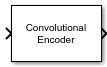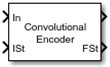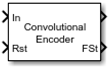# Convolutional Encoder

Encode binary data using convolutional encoding scheme

• Library:
• Communications Toolbox / Error Detection and Correction / Convolutional

Communications Toolbox HDL Support / Error Detection and Correction / Convolutional

•## Description

The Convolutional Encoder block encodes the input binary message by using the convolutional encoding scheme specified by a trellis structure. For more information, see Convolutional Coding.

This block can accept inputs that vary in length during simulation. For more information about variable-size signals, see the Variable-Size Signal Basics (Simulink) topic.

These icons show the optional block ports enabled.## Ports

### Input

expand all

Input message, specified as a binary column vector. This port is unnamed until a second input port is enabled. If the encoder takes K input bit streams (that is, it can receive 2K possible input symbols), the block input vector length is L×K for some positive integer L.

Example: `[1 1 0 1 0 0 1 1]` specifies the message as a binary row vector with eight elements.

Data Types: `double` | `single` | `Boolean` | `int8` | `int16` | `int32` | `uint8` | `uint16` | `uint32` | `ufix1`

Initial state of encoder registers for every frame input to the block, specified as a nonnegative integer.

#### Dependencies

To enable this port set the Operation mode parameter to ```Truncated (reset every frame)``` and select Specify initial state via input port.

Data Types: `double` | `uint32`

Reset state of encoder registers, specified as scalar value. Any nonzero value forces a reset of the encoder registers.

#### Dependencies

To enable this port set the Operation mode parameter to ```Reset on nonzero input via port```.

Data Types: `double` | `Boolean`

### Output

expand all

Convolutionally encoded codeword, returned as a binary column vector. This port is unnamed on the block icon. If the encoder produces N output bit streams (that is, it can produce 2N possible output symbols), the block output vector length is L×N for some positive integer L. This output inherits its data type from the `In` input.

Data Types: `double` | `single` | `Boolean` | `int8` | `int16` | `int32` | `uint8` | `uint16` | `uint32` | `ufix1`

Final state of encoder registers for every frame output from the block, returned as a nonnegative integer.

#### Dependencies

This parameter appears only when you set the Operation mode parameter to `Continuous`, ```Truncated (reset every frame)```, or ```Reset on nonzero input via port``` and you select the Output final state parameter.

Data Types: `double`

## Parameters

expand all

Trellis description of the convolutional code, specified as a structure that contains the trellis description for a rate KN code. K is the number of input bit streams, and N is the number of output bit streams.

You can either use the `poly2trellis` function to create the trellis structure or create it manually. For more about this structure, see Trellis Description of a Convolutional Code and the `istrellis` function.

The trellis structure contains these fields.

Number of symbols input to the encoder, specified as an integer equal to 2K, where K is the number of input bit streams.

Data Types: `double`

Number of symbols output from the encoder, specified as an integer equal to 2N, where N is the number of output bit streams.

Data Types: `double`

Number of states in the encoder, specified as a power of 2.

Data Types: `double`

Next states for all combinations of current states and current inputs, specified as a matrix of integers. The matrix size must be `numStates` by 2K.

Data Types: `double`

Outputs for all combinations of current states and current inputs, specified as a matrix of octal numbers. The matrix size must be `numStates` by 2K.

Data Types: `double`

Termination method of the encoded frame, specified as one of these mode values.

• `Continuous` — The block retains the encoder states at the end of each input for use with the next frame.

• `Truncated (reset every frame)` — The block treats each input independently. At the start of each input frame, the encoder states are reset to all-zeros state, or if you select Specify initial state via input port, to the state specified by the `ISt` port.

• `Terminate trellis by appending bits` — The block treats each input independently. For each input frame, extra bits are used to set the encoder states to all-zeros state at the end of the frame. The output length is given by y = N × (x + s) / K, where x is the number of input bits, and s = constraint length – 1 (or, in the case of multiple constraint lengths, s =sum(constraint length(i) – 1)).

Note

This block works for cases K ≥ 1, where it has the same values for constraint lengths in each input stream. For example, constraint lengths of [2 2] or [7 7] will work, but [5 4] will not.

• `Reset on nonzero input via port` — The block has an additional input port, labeled `Rst`. When the `Rst` input is nonzero, the encoder resets to the all-zeros state.

Note

When this block outputs sequences that vary in length during simulation and you set the Operation mode to `Truncated (reset every frame)` or `Terminate trellis by appending bits`, the block's state resets at every input time step.

Select this parameter to reset the block after computing the encoded data. The delay in the reset action allows the block to support HDL code generation. Generating HDL code, requires HDL Coder™ software.

#### Dependencies

This parameter appears only when you set the Operation mode parameter to ```Reset on nonzero input via port```.

Select this parameter to add the `ISt` input port to the block.

#### Dependencies

This parameter appears only when you set the Operation mode parameter to ```Truncated (reset every frame)```.

Select this parameter to add the `FSt` output port to the block.

#### Dependencies

This parameter appears only when you set the Operation mode parameter to `Continuous`, ```Truncated (reset every frame)```, or ```Reset on nonzero input via port```.

Select this parameter to view and enable the Puncture vector parameter.

Puncture pattern, specified as vector. The puncture vector is a pattern of `1`s and `0`s where the `0`s indicate the bits punctured in the output encoded data. The length of the vector must be an integer divisor of `length(In)`, the input message vector length.

For some commonly used puncture patterns for specific rates and polynomials, see the Yasuda , Haccoun , and Begin  references.

#### Dependencies

This parameter appears only when you select the Puncture code parameter.

## Block Characteristics

 Data Types `Boolean` | `double` | `fixed pointa` | `integer` | `single` Multidimensional Signals `no` Variable-Size Signals `yes` a ufix(1) only.